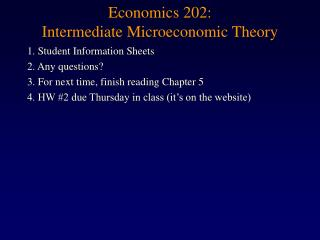# Economics 202: Intermediate Microeconomic Theory - PowerPoint PPT PresentationDownload PresentationEconomics 202: Intermediate Microeconomic Theory

Economics 202: Intermediate Microeconomic TheoryDownload Presentation## Economics 202: Intermediate Microeconomic Theory

- - - - - - - - - - - - - - - - - - - - - - - - - - - E N D - - - - - - - - - - - - - - - - - - - - - - - - - - -
##### Presentation Transcript

1. Economics 202: Intermediate Microeconomic Theory 1. Student Information Sheets 2. Any questions? 3. For next time, finish reading Chapter 5 4. HW #2 due Thursday in class (it’s on the website)

2. Budget Constraints • Shifts in the Budget Line • Change in Income • Change in Prices • Double I, Triple PX & PY? Yo-yos I/PY 2I/3PY • NB: slope measures the real price, the purchasing power of one good in terms of the other • so if both prices rise by the same % (or fall by same %), their ratio is the same  the slope of budget line stays same! • Composite consumption good x2: p1x1 + 1x2 = m • What if the price changes with quantity purchased? 2I/3PX I/PX Xylophones

3. Budget Constraints • Change in Income • lump-sum tax or subsidy (grant) • “earmarked” grant • Change in Prices • Per-unit tax or subsidy (quantity tax or subsidy) • Ad valorem tax or subsidy (value/proportional tax or subsidy)

4. Optimal Consumption • Two criteria: (1) slope of IC = slope of budget line (2) we have to be on the budget line • This will give us 2 equations in 2 unknowns, and we can solve for optimal values • General Lagrangian & MRS = PX/PY is only a necessary condition for utility maximization. Assumption of dim. MRS (strict convexity) gives sufficiency for utility maximization. • U = GT Income = \$100 Ptennis racquet = \$10 Pgatorade = \$5 • What is Roger’s optimal consumption bundle of Gatorade and tennis racquets? • Three approaches

5. Optimal Consumption • Approach #1 • Write down & solve the MRS condition and budget constraint • Approach #2 • Create unconstrained utility maximization problem • Approach #3 • Constrained utility maximization problem (use the Lagrangian) •  has an economic interpretation • Check  • Let I = \$101 and calculate the resulting increase in utility … • We had U = GT, I = \$100, PG = \$5 per bottle, PT = \$10 per racquet • This gave us G* = 10 bottles, T* = 5 tennis racuets for U* = 50 “utils”

6. Pretzels a U2 U1 U0 Final four tickets Exception: Corner Solution • At point “a”, MRS < slope of the budget line • But that is our final point since we can’t consume less than 0 Final Four tickets • NB: the optimality condition (MRS = slope of budget line) only holds for cases in which we consume positive amounts of BOTH goods. • FOC’s must be modified with a  sign, rather than = sign. • When, e.g., U/X - PX < 0, then X* = 0. • PX > MUX /  which says ?

7. Optimal Consumption • Derive the demand functions for the quasi-linear function U(X,Y) = ln X + Y X* = dX(PX, PY, I; tastes) Y* = dY(PX, PY, I; tastes) • Green is a foreshadowing to emphasize now that this is Marshallian demand (uncompensated demand) which holds income fixed. Numerical example: U = ln X + Y Income = \$10 PX = PY = \$1 • Are these homothetic preferences? • What is optimal consumption bundle (X*,Y*)? • What is utility at the optimum? • What is the marginal utility of income at the optimum?